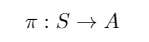04. Reinforcement Learning (Move 37): Sensor Networks

A summary of the concepts discussed in the the Sensor Networks video for a reinforcement learning course from the School of AI called Move 37. See my previous post here for the Markov Decision Processes lecture notes summary. The focus of this lecture was on the application of reinforcement learning to Sensor Networks. Although the lecture focused on the application to sensor networks, here I will focus on the new concepts highlighted in the video.

Example: Sensor Network

Objective Statement: Find the most efficient data routing strategy for a network of connected wireless devices.

Routing data from a source location to a destination has to go through intermediate nodes between the source location and destination. The objective is to find an optimal route from the source and hop between routes / nodes to reach destination node. The optimal route depends on several factors depending on the states which we will see later.

Approach

The first step is to define the problem as a Markov Decision Process:

• States (routes / nodes)
• Actions (left, right, up, down)
• Rewards (+1 if we reach the final router / node)

The ideal solution is a series of actions that need to be learned in order to complete its goal.

What needs to be learnt by the agent?

• It needs to learn how to best route data so that it reaches the right server as fast as possible.
• How to efficiently allocate energy usage amongst its nodes.
• How to react to changes in its topology.

The correct action depends on the current situation (i.e the current state), an example is if a network has really high traffic it will need to perform a different set of actions to route data than if it was low.

We will have a solution to the problem when an agent has learnt an appropriate action response to any environment state that it can observe.

Therefore we will need a Policy to give us the mapping from the environment state to the possible actions.

Types of Policies

Deterministic Policy

The most basic type of policy which maps the set of environment states to the set of possible actions. The output entirely depends on the state, which is the input.

Stochastic Policy

Lets the agent choose actions randomly. A stochastic policy is a mapping that accepts an environment state s and action a and returns the probability that the agent takes action a while in state s.

Reinforcement Learning agents learn to maximise cumulative future reward which is known as the return (R).

Types of Optimal Value Functions

The next part of the video I found rather confusing so added some comments about each section to hopefully make it more easier to understand. Any feedback to help make this easier to explain will be great.

State-value function

v_π is the state-value function for policy π. The value of state s under a policy π is:

For a given policy: for each state s, it yields the expected return (G_t) if the agent starts in state s and then uses the policy to choose its actions for all time steps.

Side comments: Essentially this means the there are no actions being chosen by the learner / agent. The given policy has control.

Action-value function

q_π is the action-value function for policy π. The value of action a in state s under a policy π is:

For a given policy: for each state s and an action a it yields the expected return if the agent starts in state s then chooses action a and then uses the policy to choose its actions for all time steps.

Side comments: This means the learner / agent has control over the initial action ‘a’ but then the policy takes over for the actions that follow.

General Comments: In the above equations we are given the policies (π). This differs to previous lectures where we had the equation to find the optimal policies (π*). The given policy here may not be the optimal policy, it was just an explanation on how to calculate the values of the state-value function and action-value function. The applications of these algorithms will probably be made clearer in next week’s lectures when applied to route planning and scheduling.

Key Question (for next lecture): How do we find the optimal action value function?

Key takeaways:

• There are two types of policies, deterministic where the action taken entirely depends on the state and stochastic which allows for randomness.
• To learn an optimal policy, we need to learn an optimal value function, of which there are 2 kinds: state-action and action-value.
• We can compute the value function using the Bellman Equation which expresses the value of any state as the sum of the immediate reward plus the value of the state that follows.

The next summary will be on the reinforcement learning from Week 2 of Move37 course. If you would like post updates follow me here and/or on Twitter. Feedback and comments are also appreciated.

Source: Deep Learning on Medium